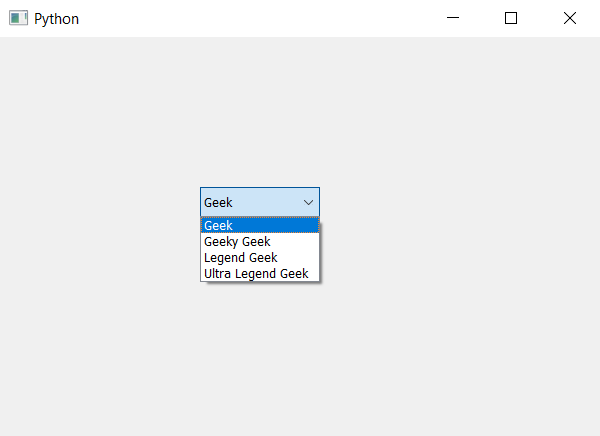Open In App

# PyQt5 – How to add multiple items to the ComboBox ?

In this article we will see how we can add multiple items to the combo box at single time. We know we can add item to the combo box with the help of addItem method but this method add only single item at a time. In order to add multiple items at a single time we have to use addItems method which will add all the items at once instead of one by one.

Argument : It takes list as argument
Action performed : It will add all the list item to the combo box

Below is the implementation –

## Python3

 `# importing libraries``from` `PyQt5.QtWidgets ``import` `*``from` `PyQt5 ``import` `QtCore, QtGui``from` `PyQt5.QtGui ``import` `*``from` `PyQt5.QtCore ``import` `*``import` `sys`  `class` `Window(QMainWindow):` `    ``def` `__init__(``self``):``        ``super``().__init__()` `        ``# setting title``        ``self``.setWindowTitle(``"Python "``)` `        ``# setting geometry``        ``self``.setGeometry(``100``, ``100``, ``600``, ``400``)` `        ``# calling method``        ``self``.UiComponents()` `        ``# showing all the widgets``        ``self``.show()` `    ``# method for widgets``    ``def` `UiComponents(``self``):` `        ``# creating a combo box widget``        ``combo_box ``=` `QComboBox(``self``)` `        ``# setting geometry of combo box``        ``combo_box.setGeometry(``200``, ``150``, ``120``, ``30``)` `        ``# geek list``        ``geek_list ``=` `[``"Geek"``, ``"Geeky Geek"``, ``"Legend Geek"``, ``"Ultra Legend Geek"``]` `        ``# adding list of items to combo box``        ``combo_box.addItems(geek_list)` `# create pyqt5 app``App ``=` `QApplication(sys.argv)` `# create the instance of our Window``window ``=` `Window()` `# start the app``sys.exit(App.``exec``())`

Output :